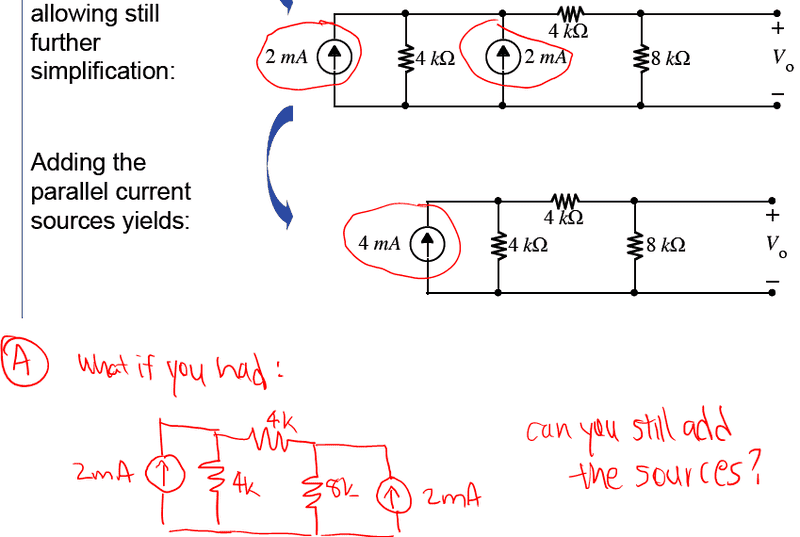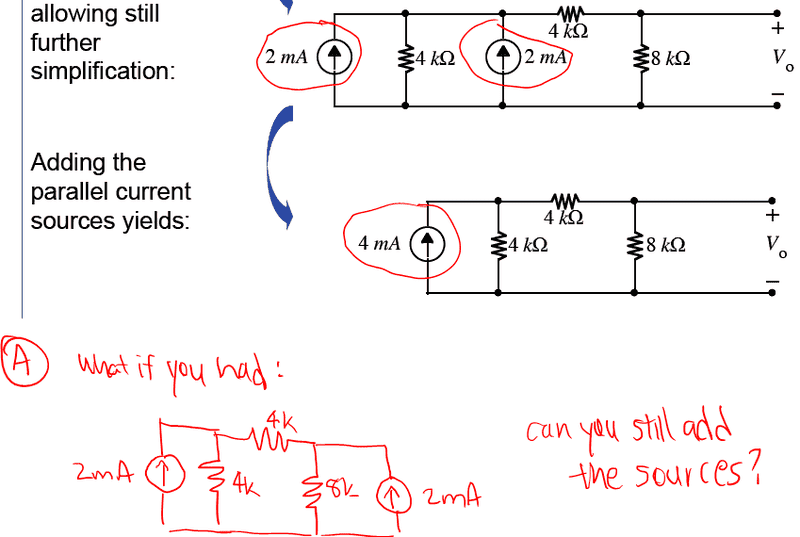# Adding parallel current sources?

## Homework Statement

I am somewhat confused about when you can add parallel current sources:Are the sources in the diagram I drew - A - in parallel? To me they look like they are but I am not sure... Is there an easy way to visualize these problems?

ehild
Homework Helper

## Homework Statement

I am somewhat confused about when you can add parallel current sources:Are the sources in the diagram I drew - A - in parallel? To me they look like they are but I am not sure... Is there an easy way to visualize these problems?

They are not. Parallel connection of either resistors or sources means that they have two pairs of terminals in common. There is a resistor connected between the upper terminals of the sources in figure A, so those terminals are not common.

ehild

PeterO
Homework Helper

## Homework Statement

I am somewhat confused about when you can add parallel current sources:Are the sources in the diagram I drew - A - in parallel? To me they look like they are but I am not sure... Is there an easy way to visualize these problems?

Your diagram has the power supply missing, but assuming it is meant to be on the right - as in the original - you cannot just combine the two like you could in the first.

To redraw, I could imagine the V- is pulled ot to the extreme left, while the V+ is pulled to the right, then each component arranged horizontal.

In case 1:

I would then have:

V- on the left, with the wire from it splitting in to two branches.
The bottom branch has the 8kΩ resistor then meets a coming together leading to the V+ on the right.
The upper branch first splits into 3 branches - with a diode, a 4kΩ and a diode on those branches. those branches come back together to a single wire which passes through a 4kΩ resistor before rejoining the bottom branch and thence to V+

The three components, shown on the left of the original circuit diagram, and now clearly seen to be in parallel with each other. Through that three-some flows 2 mA, 2mA plus what ever gets through the 4kΩ resistor - with all that current going on to flow through the second 4kΩ resistor. That mans the 2 x 2mA currents could be considered as a single 4mA current.

In case 2, if you re-draw it in a similar way the circuit would appear as 3 overall branches, with the top branch splitting into two before rejoining and passing through a 4kΩ resistor.

In that case the two diodes are completely separated, and you cannot add the 2 x 2mA to form a single 4mA

CWatters
Science Advisor
Homework Helper
Gold Member
You can only add current sources when they are connected in parallel between the same nodes. In the red circuit "A" they aren't connected to the same nodes.

To solve the red circuit "A" I would note that there are two loops one on the left and one on the right of the circuit. So it's easy to work out the voltage on the vertical resistors.

Vleft = 0.002 x 4000 = 8V

Vright = 0.002 x 8000 = 16V

So voltage drop across middle horizontal 4K resistor is 16-8 = 8V